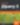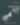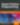# 5 jQuery.each() Function Examples

JavaScript

Share:

#### Learning jQuery 3 Fifth Edition🤓 All the power of JavaScript in a simpler format

This is an extensive overview of the `jQuery.each()` function — one of jQuery’s most important and most used functions. In this article, we’ll find out why and take a look at how you can use it.

## What is jQuery.each()

jQuery’s each() function is used to loop through each element of the target jQuery object — an object that contains one or more DOM elements, and exposes all jQuery functions. It’s very useful for multi-element DOM manipulation, as well as iterating over arbitrary arrays and object properties.

In addition to this function, jQuery provides a helper function with the same name that can be called without having previously selected or created any DOM elements.

## jQuery.each() Syntax

Let’s see the different modes in action.

The following example selects every `<div>` element on a web page and outputs the index and the ID of each of them:

``````// DOM ELEMENTS
\$('div').each(function(index, value) {
console.log(`div\${index}: \${this.id}`);
});
``````

A possible output would be:

``````div0:header
div1:main
div2:footer
``````

This version uses jQuery’s `\$(selector).each()` function, as opposed to the utility function.

The next example shows the use of the utility function. In this case the object to loop over is given as the first argument. In this example, we’ll show how to loop over an array:

``````// ARRAYS
const arr = [
'one',
'two',
'three',
'four',
'five'
];

\$.each(arr, function(index, value) {
console.log(value);
// Will stop running after "three"
return (value !== 'three');
});

// Outputs: one two three
``````

In the last example, we want to demonstrate how to iterate over the properties of an object:

``````// OBJECTS
const obj = {
one: 1,
two: 2,
three: 3,
four: 4,
five: 5
};

\$.each(obj, function(key, value) {
console.log(value);
});

// Outputs: 1 2 3 4 5
``````

This all boils down to providing a proper callback. The callback’s context, `this`, will be equal to its second argument, which is the current value. However, since the context will always be an object, primitive values have to be wrapped:

``````\$.each({ one: 1, two: 2 } , function(key, value) {
console.log(this);
});

// Number { 1 }
// Number { 2 }
``````

`

This means that there’s no strict equality between the value and the context.

``````\$.each({ one: 1 } , function(key, value) {
console.log(this == value);
console.log(this === value);
});

// true
// false
``````

`

The first argument is the current index, which is either a number (for arrays) or string (for objects).

## 1. Basic jQuery.each() Function Example

Let’s see how the jQuery.each() function helps us in conjunction with a jQuery object. The first example selects all the `a` elements in the page and outputs their `href` attribute:

``````\$('a').each(function(index, value){
console.log(this.href);
});
``````

The second example outputs every external `href` on the web page (assuming the HTTP(S) protocol only):

``````\$('a').each(function(index, value){

}
});
``````

``````<a href="https://www.sitepoint.com/">SitePoint</a>
<a href="https://developer.mozilla.org">MDN web docs</a>
<a href="http://example.com/">Example Domain</a>
``````

The second example would output:

``````https://www.sitepoint.com/
https://developer.mozilla.org/
http://example.com/
``````

We should note that DOM elements from a jQuery object are in their “native” form inside the callback passed to `jQuery.each()`. The reason is that jQuery is in fact just a wrapper around an array of DOM elements. By using `jQuery.each()`, this array is iterated in the same way as an ordinary array would be. Therefore, we don’t get wrapped elements out of the box.

With reference to our second example, this means we can get an element’s `href` attribute by writing `this.href`. If we wanted to use jQuery’s `attr()` method, we would need to re-wrap the element like so: `\$(this).attr('href')`.

## 2. jQuery.each() Array Example

Let’s have another look at how an ordinary array can be handled:

``````const numbers = [1, 2, 3, 4, 5];
\$.each(numbers, function(index, value){
console.log(`\${index}: \${value}`);
});
``````

This snippet outputs:

``````0:1
1:2
2:3
3:4
4:5
``````

Nothing special here. An array features numeric indices, so we obtain numbers starting from 0 and going up to N-1, where N is the number of elements in the array.

## 3. jQuery.each() JSON Example

We may have more complicated data structures, such as arrays in arrays, objects in objects, arrays in objects, or objects in arrays. Let’s see how `jQuery.each()` can help us in such scenarios:

``````const colors = [
{ 'red': '#f00' },
{ 'green': '#0f0' },
{ 'blue': '#00f' }
];

\$.each(colors, function() {
\$.each(this, function(name, value) {
console.log(`\${name} = \${value}`);
});
});
``````

This example outputs:

``````red = #f00
green = #0f0
blue = #00f
``````

We handle the nested structure with a nested call to `jQuery.each()`. The outer call handles the array of the variable `colors`; the inner call handles the individual objects. In this example each object has only one key, but in general, any number could be tackled with this code.

## 4. jQuery.each() Class Example

This example shows how to loop through each element with assigned class `productDescription` given in the HTML below:

``````<div class="productDescription">Red</div>
<div>Pink</div>
<div class="productDescription">Orange</div>
<div class="generalDescription">Teal</div>
<div class="productDescription">Green</div>
``````

We use the `each()` helper instead of the `each()` method on the selector.

``````\$.each(\$('.productDescription'), function(index, value) {
console.log(index + ':' + \$(value).text());
});
``````

In this case, the output is:

``````0:Red
1:Orange
2:Green
``````

We don’t have to include index and value. These are just parameters that help determine which DOM element we’re currently iterating on. Furthermore, in this scenario we can also use the more convenient `each` method. We can write it like this:

``````\$('.productDescription').each(function() {
console.log(\$(this).text());
});
``````

And we’ll obtain this on the console:

``````Red
Orange
Green
``````

Note that we’re wrapping the DOM element in a new jQuery instance, so that we can use jQuery’s `text()` method to obtain the element’s text content.

## 5. jQuery.each() Delay Example

In the next example, when the user clicks the element with the ID `5demo` all list items will be set to orange immediately.

``````<ul id="5demo">
<li>One</li>
<li>Two</li>
<li>Three</li>
<li>Four</li>
<li>Five</li>
</ul>
``````

After an index-dependent delay (0, 200, 400, … milliseconds) we fade out the element:

``````\$('#5demo').on('click', function(e) {
\$('li').each(function(index) {
\$(this).css('background-color', 'orange')
.delay(index * 200)
});

e.preventDefault();
});
``````

## Conclusion

In this post, we’ve demonstrated how to use the `jQuery.each()` function to iterate over DOM elements, arrays and objects. It’s a powerful and time-saving little function that developers should have in their toolkits.

And if jQuery isn’t your thing, you might want to look at using JavaScript’s native Object.keys() and Array.prototype.forEach() methods. There are also libraries like foreach which let you iterate over the key value pairs of either an array-like object or a dictionary-like object.

Remember: `\$.each()` and `\$(selector).each()` are two different methods defined in two different ways.

This popular article was updated in 2020 to reflect current best practices and to update the conclusion’s advice on native solutions using modern JavaScript. For more in-depth JavaScript knowledge, read our book, JavaScript: Novice to Ninja, 2nd Edition.### Florian Rappl

Florian Rappl is an independent IT consultant working in the areas of client / server programming, High Performance Computing and web development. He is an expert in C/C++, C# and JavaScript. Florian regularly gives talks at conferences or user groups. You can find his blog at florian-rappl.de.### James Hibbard

Currently I work for SitePoint as editor of their JavaScript hubs and technical editor for various books (e.g. JavaScript: Novice to Ninja and Jump Start Vue.js). I also work as a network admin and freelance web dev, where I spend a fair bit of my time working on Rails apps.

#### New books out now!Learn how Git works, and how to use it to streamline your workflow!Google, Netflix and ILM are Python users. Maybe you should too?